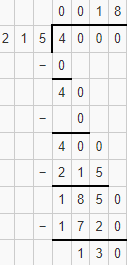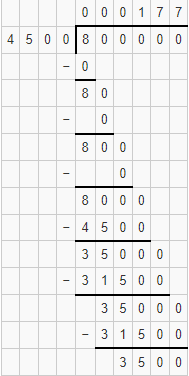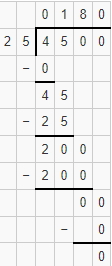### Chapter - 1 Knowing Our Numbers

Q
##### Knowing Our Numbers

Question:

1. A book exhibition was held for four days in a school. The number of tickets sold at the counter on the first, second, third and final day was respectively 1094, 1812, 2050 and 2751. Find the total number of tickets sold on all the four days.

Solution:

Number of tickets sold on 1st day = 1094

Number of tickets sold on 2nd day = 1812

Number of tickets sold on 3rd day = 2050

Number of tickets sold on 4th day = 2751

Hence, number of tickets sold on all the four days = 1094 + 1812 + 2050+ 2751 = 7707 tickets

2. Shekhar is a famous cricket player. He has so far scored 6980 runs in test matches. He wishes to complete 10,000 runs. How many more runs does he need?

Solution:

Shekhar scored = 6980 runs

He want to complete = 10000 runs

More runs need to score to complete 10000 runs = 10000 - 6980 = 3020

Hence, he need 3020 more runs to score

3. In an election, the successful candidate registered 5,77,500 votes and his nearest rival secured 3,48,700 votes. By what margin did the successful candidate win the election?

Solution:

No. of votes secured by the successful candidate = 5,77,500

No. of votes secured by his rival = 3,48,700

Margin by which he won the election = 577500 - 348700 - 228800 votes

The successful candidate won the election by 228800 votes.

4. Kirti bookstore sold books worth  ₹ 2,85,891 in the first week of June and books worth  4,00,768 in the second week of the month. How much was the sale for the two weeks together? In which week was the sale greater and by how much?

Solution:

Price of books sold in June first week =  285891

Price of books sold in June second week =  400768

No. of books sold in both weeks together =  285891 +  400768 =  686659 The sale of books is the highest in the second week

The difference in the sale in both weeks = ₹ 400768 -  285891 =  114877

The sale in the second week was greater by  114877 than in the first week.

5. Find the difference between the greatest and the least 5-digit number that can be written using the digits 6, 2, 7, 4, 3 each only once.

Solution: Given digits are 6, 2, 7, 4, 3.

The greatest 5 digit number that can be formed by given digits is 76432.

The least 5 digit number that can be formed by given digits is 23467.

Difference between the two numbers = 76432 - 23467 = 52965.

6. A machine, on an average, manufactures 2,825 screws a day. How many screws did it produce in the month of January 2006?

Solution:

Number of screws manufactured in a day = 2825

Since January month has 31 days

Hence, the number of screws manufactured in January = 31 x 2825 = 87575

Hence, the machine produces 87575 screws in the month of January 2006.

7. A merchant had ₹ 78,592 with her. She placed an order for purchasing 40 radio sets at ₹ 1200 each. How much money will remain with her after the purchase?

Solution:

Total money the merchant had =  78592

Number of radio sets she placed an order for purchasing = 40 radio sets

Cost of each radio set =  1200

So, cost of 40 radio sets =  1200 x 40 =  48000

Money left with the merchant =  78592 -  48000 =  30592

Hence, money left with the merchant after purchasing radio sets is ₹ 30592.

8. A student multiplied 7236 by 65 instead of multiplying by 56. By how much was his answer greater than the correct answer? (Hint: Do you need to do both the multiplications?)

Solution:

Difference between 65 and 56 = 65 - 56 = 9

The difference between the correct and incorrect answer = 7236 x 9 = 65124

Hence, by 65124, the answer was greater than the correct answer.

9. To stitch a shirt, 2 m 15 cm cloth is needed. Out of 40 m cloth, how many shirts can be stitched and how much cloth will remain? (Hint: convert data in cm.)

Solution:

Total length of the cloth = 40 m {Given}

Total length of the cloth in cm = 40 x 100 cm = 4000 cm

Cloth required to stitch one shirt = 2 m 15 cm

Cloth required to stitch one shirt in cm = 2 x 100 + 15 cm = 215 cm

Number of shirts that can be stitched out of 4000 cm = 4000/215Hence 18 shirts can be stitched out of 40 m and 130 cm of cloth is left out.

10. Medicine is packed in boxes, each weighing 4 kg 500g. How many such boxes can be loaded in a van which cannot carry beyond 800 kg?

Solution:

Weight of one box = 4 kg 500 g = 4 x 1000 + 500 = 4500 g

Maximum weight carried by the van 800 kg = 800 × 1000 = 800000

The number of boxes that can be loaded in the van = 800000  4500Hence, 177 boxes can be loaded in the van.

11. The distance between the school and a student’s house is 1 km 875 m. Everyday she walks both ways. Find the total distance covered by her in six days.

Solution:

Distance covered between school and house = 1 km 875 m = 1000 + 875 = 1875 m

Since the student walks both ways.

Hence, distance travelled by the student in one day = 2 x 1875 = 3750 m

Distance travelled by her in 6 days = 3750 m x 6 = 22500 m = 22 km 500 m

Total distance covered by the student in six days is 22 km and 500 m.

12. A vessel has 4 litres and 500 ml of curd. In how many glasses, each of 25 ml capacity, can it be filled?

Solution:

Quantity of curd in the vessel = 4 l 500 ml = 4 x 1000 + 500 = 4500 ml

Capacity of 1 glass = 25 ml

Number of glasses that can be filled with curd = 4500  25Hence, 180 glasses can be filled with curd.